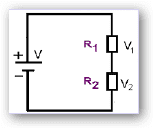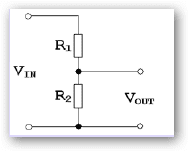# Basic Electronics – Resistor Combinations

## RESISTOR COMBINATIONS

• When we do not get specific resistor values we have to either use variable resistors such as potentiometers or presets to obtain such precise values. Pots are too expensive to use forevery case.
• Another scheme is to combine two or more resistors to obtain the necessary precise values.Such resistor combinations can cost as little as 50p or so only.
• Then the question arises as to how one should combine these resistors, because, they can be combined in two different ways.
• These are called “Series” and “Parallel” combinations.

## Series Combinations

R Total= R1+ R2

• Calculating values for two or more resistors in series is simple, add all the values up.• The connection ensures that the SAME current flows through all resistors.
• In this type of connection RT will always be GREATER than any of the included resistors.

Even if we have more than two resistors the total resistance is the sum of all the resistors connected in series:

R Total= R1+ R2+ R3 +•••••

• Total Applied voltage is divided by two resistors
• Current in the circuit is  I = V/(R1+R2)
• Voltage across R1 and R2 are from OHMS law.

V1= I*R1

V2=I* R2

Total voltage V=V1+V2

For Example if V=12v and the 2 resistors are 1k each, then the current in the circuit is

12/2k=6mA

The voltage across each resistor is 6v

Thus the series combination is characterized by

• The same current flows through all the resistors connected in series.
• The resultant resistor is SUM of all the resistors in series
• Series resistors divide the total voltage proportional to their magnitude.

## Resistors in Parallel

In Parallel combination, 2 paths are available for current, hence the current divides but the voltage across the resistors is same.

1/R total =1/R1 + 1/R2   or

R total = (R1*R2) / R1 + R2• If the two resistors are equal, the current will divide equally and total resistance will be exactly half.
• For example if voltage is 12v and there are 2 resistance for 1k each,

The current through each resistance will be 12v/1k= 12 mA. Hence the total current is 12 mA.

Effective resistance is 0.5k

Thus the parallel connection is characterized by

• The same voltage exists across all the resistors connected in parallel, and
• The reciprocal of resultant resistor is the sum of reciprocals of all resistors in parallel, and
• Parallel resistors divide the total current in an inverse proportion to their magnitude.

## Potential Divider

Since series resistors divide voltage, this idea can be used to get smaller voltage from a power supply output. For example, we have a power supply with 10V fixed output. But we want only 5V from it.

Vout= Vin(R2/(R+R2))

• The Current I=Vin/R1+R2
• Since the current I flows through R2, voltage developed across it from Ohm’s law is Vo=I*R2=( Vin/R1+R2) * R2Vo = (R2/R1+R2) Vi
• If R1=R2, then Vo=Vi/2

R1 and R2 cab be 100k or 100 ohm. Which one to be used?

If we need more current through load then R1 must be small. But too small a value will cause energy drain on the power supply. So the value must be chosen very carefully.

### Note:

• When two resistors are in parallel then their overall power rating is increased.
• If both resistors are the same value and same power rating, then the total power rating is doubled. If parallel resistances are not equal, then the resistors with smaller values will be required to handle more power.
• Four identical 0.25W resistors can be wired in parallel to give a resistor with one fourth the value in ohms, but four times the power rating. (1.0W). This is most useful when we require higher power handling, but don’t want to go out and buy more expensive (and physically larger) resistors.
• We have already seen earlier, that the power (in watts) can be calculated by multiplying voltage by current. P=V * I
• By using ohms law, the parallel or series resistor formulas and the above formula, a minimum power rating for a certain resistor can be calculated. If this is exceeded the resistor is likely to get hot and hopefully quietly breakdown.

This site uses Akismet to reduce spam. Learn how your comment data is processed.